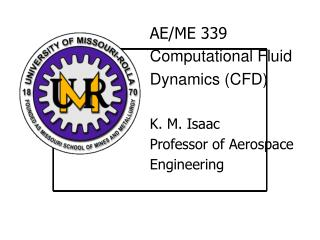DownloadDownload PresentationAE/ME 339 Computational Fluid Dynamics (CFD) K. M. Isaac Professor of Aerospace Engineering

# AE/ME 339 Computational Fluid Dynamics (CFD) K. M. Isaac Professor of Aerospace Engineering

Download Presentation## AE/ME 339 Computational Fluid Dynamics (CFD) K. M. Isaac Professor of Aerospace Engineering

- - - - - - - - - - - - - - - - - - - - - - - - - - - E N D - - - - - - - - - - - - - - - - - - - - - - - - - - -
##### Presentation Transcript

1. AE/ME 339 Computational Fluid Dynamics (CFD) K. M. Isaac Professor of Aerospace Engineering

2. Computational Fluid Dynamics (AE/ME 339) K. M. Isaac MAEEM Dept., UMR Quiz 1

3. Computational Fluid Dynamics (AE/ME 339) K. M. Isaac MAEEM Dept., UMR Test 1 topics Modeling of fluid flow in a constant area pipe with heat addition and/or friction Resulting ODE and its numerical solution use of Taylor series to approximate functions and their derivatives Numerical solution of initial value problems using R-K

4. Computational Fluid Dynamics (AE/ME 339) K. M. Isaac MAEEM Dept., UMR Unsteady 1D heat conduction equation Nature of the equation Discretization of derivatives using Taylor series Taylor series in more than one independent variable An example of a non-linear PDE from fluid mechanics Compact notation for CD approximation for 2nd derivative

5. Computational Fluid Dynamics (AE/ME 339) K. M. Isaac MAEEM Dept., UMR Explicit method of solution Problem setup Initial and boundary conditions von Neumann stability analysis for explicit and implicit schemes Solution procedure for implicit method using Gauss elimination Crank-Nicolson method. Stability of C-N ADI method Step-by-step implementation of ADI

6. Computational Fluid Dynamics (AE/ME 339) K. M. Isaac MAEEM Dept., UMR Types of boundary conditions Dirichlet, Neumann and mixed BC Non-linear PDEs. Linearization techniques

7. Computational Fluid Dynamics (AE/ME 339) K. M. Isaac MAEEM Dept., UMR